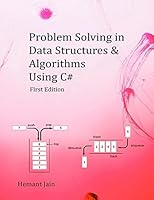# Problem Solving in Data Structures & Algorithms Using C#: Programming Interview Guide## Book Description

"Problem Solving in & Algorithms" is a series of books about the usage of Data Structures and Algorithms in programming. The book is easy to follow and is written for interview preparation point of view. In various books, the examples are solved in various languages like Go, C, C++, Java, C#, , , and PHP.

GitHub Repositories
https://github.com/Hemant-Jain-Author

Book’s Composition
This book is designed for interviews so in Chapter 0, various preparation plans are proposed. Then in chapters 1, a brief introduction of the programming language and concept of recursion is explained. A number of problems based on recursion and array are explained.

Then in the coming chapter, we will be looking into complexity analysis. Then we will be looking into Sorting & Searching techniques.

Then will look into the various data structures and their algorithms. We will be looking into a Linked List, Stack, Queue, Trees, Heap, Hash Table and .

Then we will be looking into algorithm analysis, we will be looking into Brute Force algorithms, Greedy algorithms, Divide & Conquer algorithms, Dynamic Programming, and Backtracking.

In the end, we will be looking into System Design, which will give a systematic approach for solving the design problems in an Interview.

Chapter 0: How to use this book.
Chapter 1: Introduction - Programming Overview
Chapter 2: Algorithms Analysis
Chapter 3: Approach to solve algorithm design problems
Chapter 4: Abstract Data Type
Chapter 5: Searching
Chapter 6: Sorting
Chapter 8: Stack
Chapter 9: Queue
Chapter 10: Tree
Chapter 11: Priority Queue
Chapter 12: Hash-Table
Chapter 13: Graphs
Chapter 14: String Algorithms
Chapter 15: Algorithm Design Techniques
Chapter 16: Brute Force Algorithm
Chapter 17: Greedy Algorithm
Chapter 18: Divide & Conquer
Chapter 19: Dynamic Programming
Chapter 20: Backtracking
Chapter 21: Complexity Theory
Chapter 22: Interview Strategy
Chapter 23: System Design

## Book Details

• Title: Problem Solving in Data Structures & Algorithms Using C#: Programming Interview Guide
• Author:
• Length: 475 pages
• Edition: First
• Language: English
• Publisher:
• Publication Date: 2016-11-14
• ISBN-10: 1540407306
• ISBN-13: 9781540407306